# Curvature of a triangle mesh, definition and computation.Defining and giving the formula to compute the curvature over a triangle mesh at some vertices.

## Normal curvature

By intersecting a surface with a plane we see that the section defines a curve: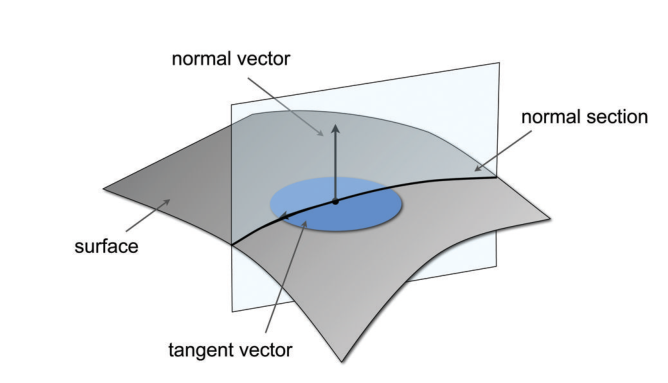The curvature of this curve is called 'normal curvature' and noted $$k_n$$ because the plane is spanned through the normal $$\boldsymbol n$$ and an arbitrary tangent vector $$\boldsymbol t$$. Now, given a point $$\boldsymbol p$$ on the surface you can find an infinity of curvatures given the direction you choose for your tangent vector $$\boldsymbol t$$.

## Principal curvatures

But how can we characterize the curvature of a surface at a given point when we seem to have an infinity of curvatures? Don't worry! It can be shown that when rotating the normal plane around $$\boldsymbol n$$ you can always find two 'principal curvatures' $$k_1$$ and $$k_2$$ respectively defining the maximal and minimal curvature at a given point $$\boldsymbol p$$ of the surface: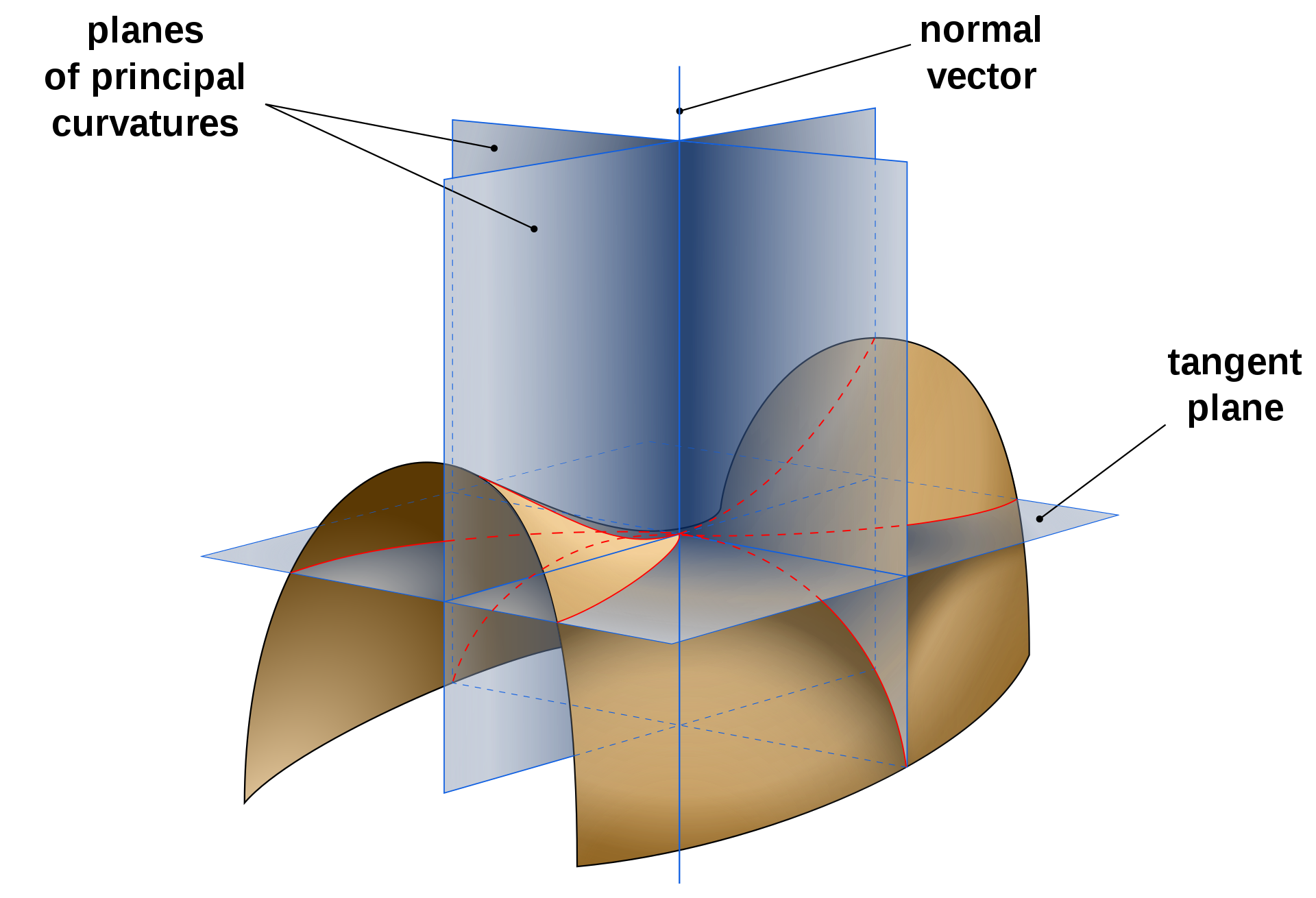In addition, the principal curvatures $$k_1$$ and $$k_2$$ are associated to the 'principal directions' $$\boldsymbol t_1$$ and $$\boldsymbol t_2$$ which are always found to be orthogonal to each other! What's more, you can define any 'normal curvature' $$k_n$$ as a combination of the principal curvatures $$k_1$$ and $$k_2$$ using Euler's theorem below:

$k_n = k_1\cos^2\theta + k_2\sin^2\theta$

Where $$\theta$$ is the angle between $$\boldsymbol t_1$$ (maximal curvature direction) and $$\boldsymbol t_n$$ the normal curvature.

### Calculation

One can compute the principal curvatures $$k_1$$ and $$k_2$$ according to the Mean curvature $$H$$ and the Gaussian curvature $$k_g$$, don't worry we will define both $$H$$ and $$k_g$$ in the next sections:

\begin{align*} k_1 & = H + \sqrt{ H^2 - k_g} \\ k_2 & = H - \sqrt{ H^2 - k_g} \end{align*}

## Gaussian curvature

The Gaussian is the product of principal curvatures $$k_g = k_1 * k_2$$ on a triangular mesh it can be computed at vertex $$\boldsymbol p_i$$ as follows:

$k_g = \frac{ 2 \pi - \sum \theta_j } { A_i }$

Where the angle $$\theta_j$$ is defined as: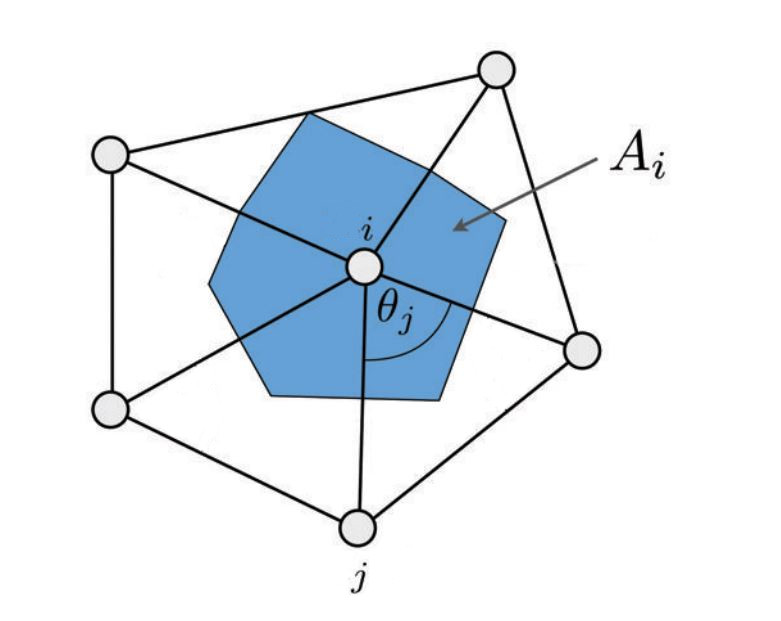$$A_i$$ can be computed as:

• 'mixed voronoi area'.
• 'barycentric cell area' (One third of the sum of the triangles' area adjacent to $$i$$): $$A_i = \frac{1}{3} {\sum\limits_{{T_j \in \mathcal N(i)}} {area(T_j)}}$$

### Interpreting the Gaussian curvature's value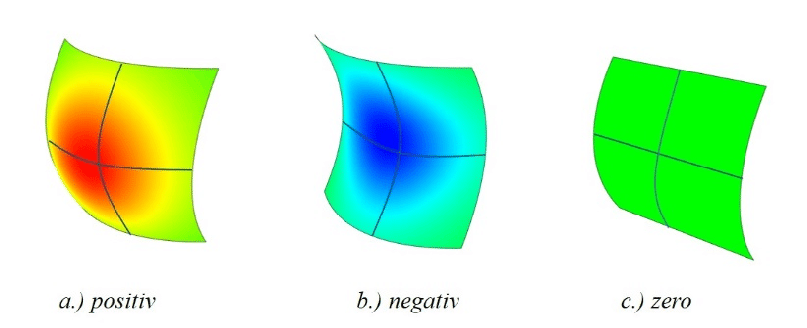It can help to locally characterize the shape:

• $$k_g > 0$$ means surface is locally a bowl-like shape. (elliptical point)
• $$k_g < 0$$ means surface is locally a saddle-like shape. (hyperbolic point)
• $$k_g = 0$$ means surface is locally flat in at least one direction (parabolic point)

## Mean curvature

The mean curvature is the average of the principal curvatures:

$$H= \frac { k_1 + k_2 } { 2 }$$

In the smooth setting the mean curvature is the average of the normal curvatures in $$[0 - 2\pi]$$

\begin{align*} k_h & = \frac{1}{2\pi} \int_0^{2\pi} { k_n(\theta) \, d\theta } \\ & \text{ recall the relationship between principal and normal curvature:} \\ k_h & = \frac{1}{2\pi} \int_0^{2\pi} { k_1\cos^2(\theta) + k_2\sin^2(\theta) \, d\theta } \\ \end{align*}

If you're lazy like me you can solve this with Maxima , just insert in Maxima's command line:

    ratsimp(1/(2*%pi)*integrate(k1*cos(t)^2 + k2*sin(t)^2, t, 0, 2*%pi));


step by step development:

\begin{align*} k_h & = \frac{1}{2\pi} \left[ \int_0^{2\pi} { k_1 \cos^2(\theta) \, d\theta } \, {\color{red}+} \int_0^{2\pi} {k_2\sin^2(\theta) \, d\theta } \right] ~~~~~~\raise 0.8em {\color{grey}\scriptsize{ \int a + b = \int a + \int b}} \\ k_h & = \frac{1}{2\pi} \left[ {\color{red}k_1} \int_0^{2\pi} { \cos^2(\theta) \, d\theta } + {\color{red}k_2} \int_0^{2\pi} {\sin^2(\theta) \, d\theta }\right] ~~~~~~\raise 0.8em {\color{grey}\scriptsize{ \int a.f(x) = a.\int f(x)}} \\ k_h & = \frac{1}{2\pi} \left[ k_1\int_0^{2\pi} { {\color{red}\frac{1+ \cos(2\theta)}{2}} \, d\theta } + k_2 \int_0^{2\pi} {{\color{red}\frac{1 - \cos(2\theta)}{2}} \, d\theta }\right] ~~~~~~\raise 0.8em {\color{grey}\scriptsize{ \href{https://en.wikipedia.org/wiki/List_of_trigonometric_identities#Power-reduction_formulae}{\text{Power reduction formulae}} }} \\ k_h & = \frac{1}{{\color{red}4}\pi} \left[ k_1 \int_0^{2\pi} { {1+ \cos(2\theta)} \, d\theta } + k_2 \int_0^{2\pi} {{1 - \cos(2\theta)} \, d\theta } \right]\\ k_h & = \frac{1}{4\pi} \left[ k_1 \int_0^{2\pi} {1\, d\theta} \, {\color{red}+} k_1 \int_0^{2\pi}{ \cos(2\theta) \, d\theta } + k_2 \int_0^{2\pi} {1\, d\theta} \,{\color{red}-} k_2 \int_0^{2\pi}{ \cos(2\theta) \, d\theta }\right] \\ k_h & = \frac{1}{4\pi} \left[ k_1 {\color{red} \left [ x \right ]_{0}^{2\pi}} + k_1 \int_0^{2\pi}{ \cos(2\theta) \, d\theta } + \cdots \right] ~~~~~~\raise 0.8em {\color{grey}\scriptsize{ \frac{d(x + c)}{dx} = 1 }} \\ k_h & = \frac{1}{4\pi} \left[ k_1 {\color{red} \left [ 2\pi - 0 \right ]} + k_1 {\color{red} \left [ \frac{\sin(2\theta)}{2} \right ]_{0}^{2\pi}} + \cdots \right] ~~~~\raise 0.8em {\color{grey}\scriptsize{ \text{chain rule:} f'(g(x)) = g'(x)f'(g(x)) \text{ so, } \frac{d}{dx} ( \frac{1}{2} \, \sin(2x) + c ) = \frac{2}{2} \, \cos(2x) }} \\ k_h & = \frac{1}{4\pi} \left[ k_1 {\color{red} 2\pi} + k_1 {\color{red} \left [ \frac{\sin(4\pi)}{2} - \frac{\sin(0)}{2} \right]} + \cdots \right]\\ k_h & = \frac{1}{4\pi} \left[ k_1 2\pi + k_1 {\color{red} 0} + \cdots \right] ~~~~~~\raise 0.8em {\color{grey}\scriptsize{ sin(0) = sin(2\pi) = sin(4\pi) = 0 }} \\ k_h & = \frac{1}{4\pi} \left[ k_1 2\pi + k_2 {\color{red} 2\pi } - k_2 {\color{red} 0} \right]\\ k_h & = \frac{1}{2} \left[ k_1 + k_2 \right] \\ \end{align*} 🥲

In addition, the mean curvature can also be expressed using the Laplace operator:

$$H = \frac{ \| \Delta p_i \|}{ 2 }$$

In other words, to find the mean curvature for a triangle mesh, we need to apply the discrete Laplace operator at some vertex $$\boldsymbol p_i$$ as follows:

$$\Delta \vec p_i = \frac{1} {2A_i} \sum\limits_{{j \in \mathcal N(i)}} { (\cot \alpha_{ij} + \cot \beta_{ij}) (p_j - p_i) }$$

• $${j \in \mathcal N(i)}$$ is the list of vertices directly adjacent to the vertex $$i$$
• $$\cot \alpha_{ij} + \cot \beta_{ij}$$ are the famous cotangent weights (a real value)
• $$p_i, p_j, ...$$ vertices position.
• $$A_i$$ is the cell area as described earlier (a real value).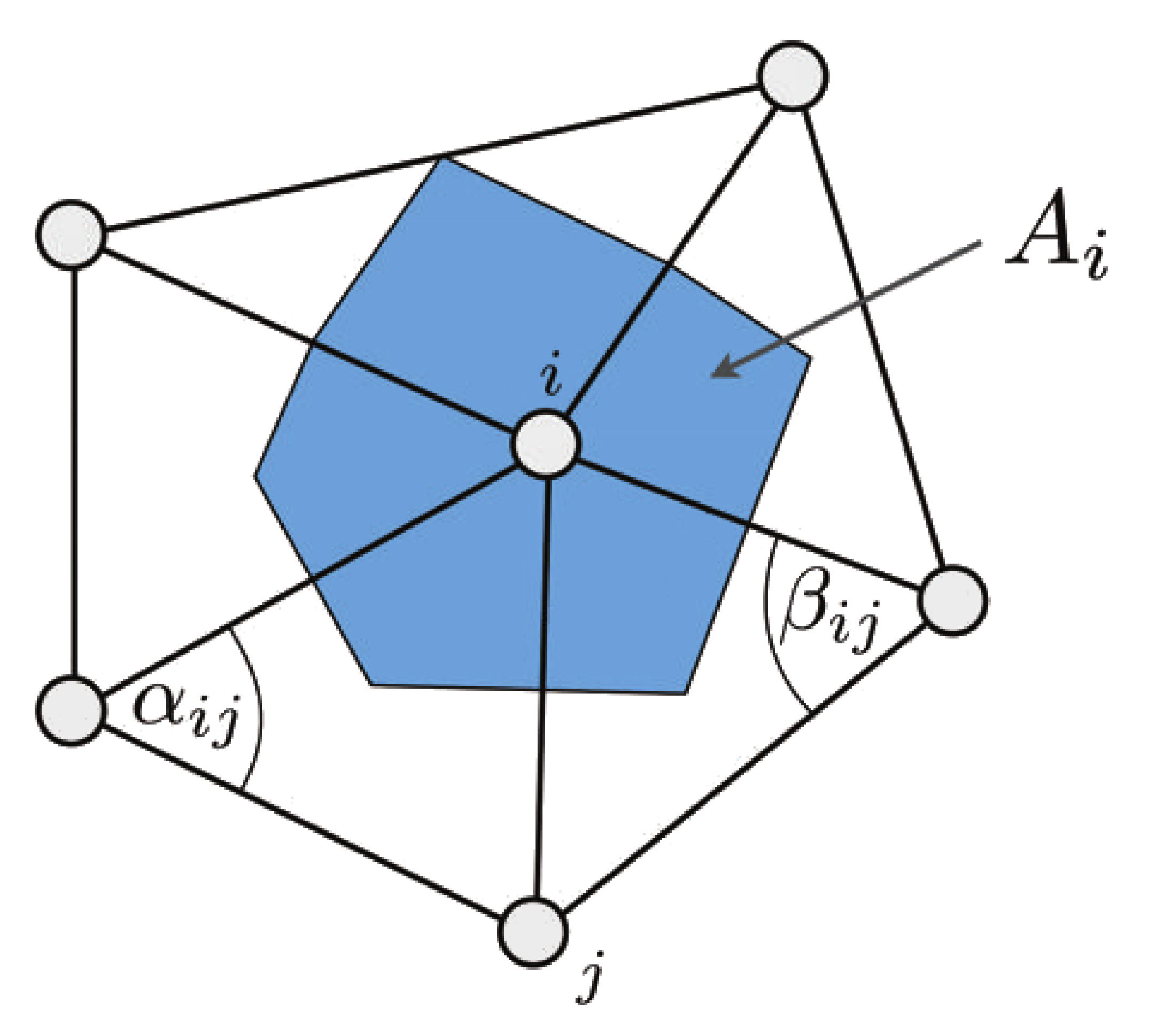### Interpreting the Mean curvature's value

It does not characterize the shape but gives the amount of curvature in one way or another:

• $$H = 0$$ -> saddle or flat surface
• $$H > 0$$ -> amount of convexity (peak)
• $$H < 0$$ -> amount of concavity (pit)

## Gaussian VS Mean curvature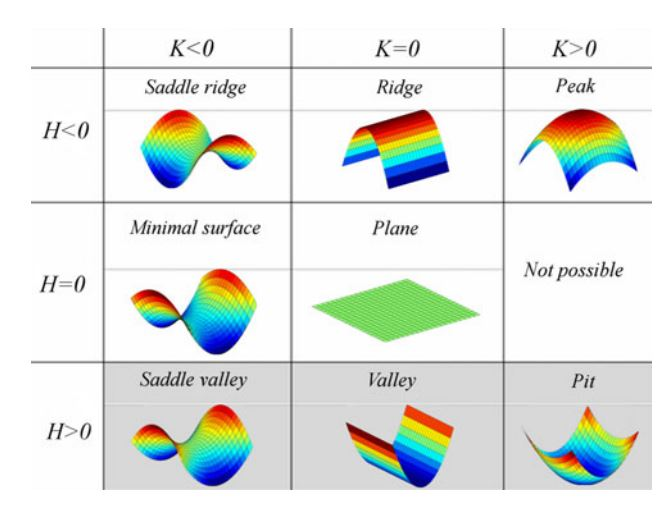## References

One comment

The ‘barycentric cell area’ is not “sum of the triangles’ area adjacent to i times 3”, but “sum of the triangles’ area adjacent to i divided by 3”

From page 10 in [http://www.geometry.caltech.edu/pubs/DMSB_III.pdf]:
“It is interesting to notice that using the barycentric area as an averaging
region results in an operator very similar to the definition of the mean cur-
vature normal by Desbrun et al. [DMSB99], since A_Barycenter is a third of the
whole 1-ring area A_1-ring used in their derivation.”
——————————
Rodolphe: Nice catch, thank you!

Felix - 10-10-’22 21:51
(optional field, I won't disclose or spam but it's necessary to notify you if I respond to your comment)
All html tags except <b> and <i> will be removed from your comment. You can make links by just typing the url or mail-address.
Anti-spam question: